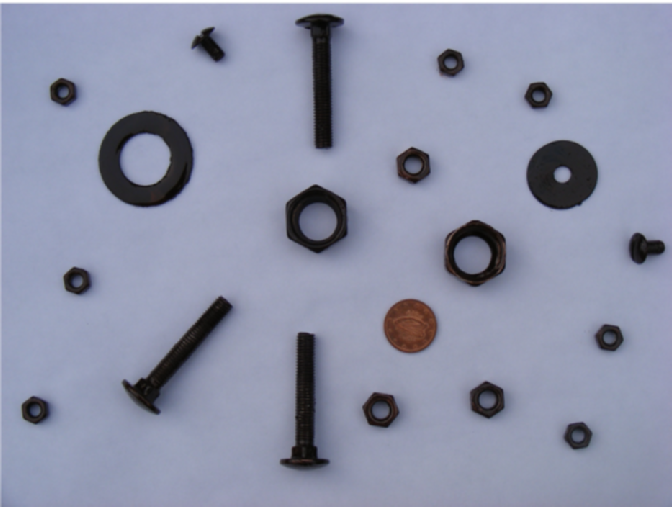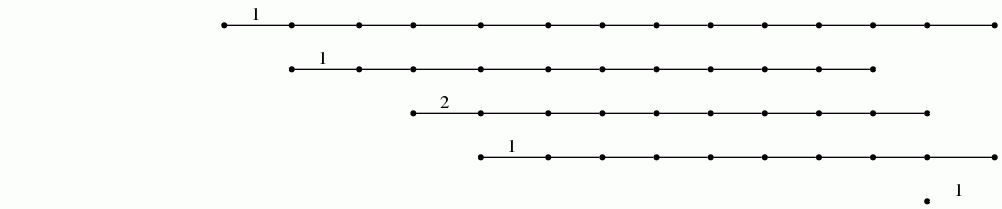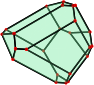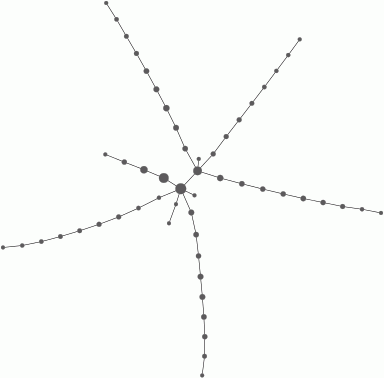## Covid-19 update

From 6pm March 12 the lectures, continuous assessment, and summer examination for this module will be delivered online.

• #### Graham's remaining lectures

Slides for Graham's lectures 19, 20, 21, 22, 23, 24 will be uploaded, as usual, shortly after the lecture slot. A recording of Graham delivering the lecture, to an empty class, will also be uploaded.
• #### Emil's remaining lectures

Details to be announced.
• #### Remaining homeworks

The fourth homework should be submitted by 23 March. The fifth homework should be submitted by 3 April
• #### Semester exams

The plan is to deliver our Maths exams more or less as usual. They'll be made available on Blackboard at the time scheduled in the official exam timetable. Students will answer them at home. They'll be given two hours to write out the solutions. Then they'll be given a short period of time (30 mins say) to scan or photograph the answers and upload them to Blackboard. The onus will be on the student to make sure that the uploaded file is easily readable by the grader.

## Module Content

Data analysis is a process of inspecting, cleansing, transforming, and modeling data with the goal of discovering and communicating useful information, informing conclusions, and supporting decision-making. Data analysis involves many overlapping areas of study, such as descriptive statistics (concerned with summarizing information in a sample), statistical inference (concerned with inferences about a population based on properties of a sample), machine learning (concerned with performing tasks using algorithms that rely on patterns and inference), data mining (sometimes considered a subfield of machine learning and concerned with discovering patterns in large data sets), information visualization (concerned with the visual representations of abstract data to reinforce human cognition), and several other areas. See this interesting review for comments about the related term data science.

Geometry is concerned with questions of shape, size, relative position of figures, isometry, and the properties of space. It underlies much of data analysis, as can be seen from textbooks such as those by [Kendall], [Le Roux], [Kirby], [Tossdorff], [Hartmann], [Outot], [Tierny], [Edelsbrunner], [Patrangenaru], [Biau], [Wichura], [Dryden]. The recent online textbook Mathematical Foundations for Data Analysis by Jeff M. Phillips again emphasizes the importance of a geometric understanding of techniques when applying them to data analysis.

This module focuses on some geometric methods used in data analysis. It covers the geometric and algorithmic aspects of these methods, as well as their implementation as Python code on Linux computers, and their application to a range of different types of data. The first half of the course emphasizes geometric aspects of classical techniques such as least squares fitting, principal component analysis, hierarchical clustering, nearest neighbour searching, and the Johnson-Lindenstrauss Theorem for dimensionality reduction. The second half of the course covers more recent techniques that have been developed over the last two or three decades, and emphasizes topological aspects as well as geometric aspects. The second half of the course makes use of R interfaces to Python Mapper and to efficient C++ routines for persistent homology.

Part I = CS4102: Classical Techniques (5 ECTS, first 24 lectures)
• Least Squares Fitting
• Principal Component Analysis
• Hierarchical Clustering and Persistence
• Nearest Neighbours and the Johnson–Lindenstrauss Theorem
Part II = CS4102: Topological Data Analysis (5 ECTS, second 24 lectures)
• Topological Preliminaries
• Mapper Clustering
• Persistent Homology

## Module Assessment

Part I will be assessed by a 2-hour written exam (52%) and three continuous assessment assignments (16% each).

Part II will be assessed by a 2-hour written exam (50%) and two continuous assessment assignments (25% each).

Each exam will consist of four questions, with full marks for four correct answers.

Each assignment will consist of a data analysis problem that needs to be tackled using the Python programming language or the R programming environment, and submitted (by email to both lecturers) as a PDF document.

## Emil's Lecture Notes

These will be posted here.

## Exam Details

Exam hints/help for MA500/CS4102/CS4103 exams can be found here and here.

## Lecture Notes

Lecture Notes

Lecture Summaries
1
Began by explaining the terms "geometry", "data analysis", "statistics", "probability". Then explained how to find the line y=b0 + b1 x that "best fits" a collection of data points (xi,yi) for i=1,2,...,n. We took "best fit" to mean the line that minimizes the sum of the squares of the residuals ei = yi - b0 - b1xi .
2
Explained how to determine the best (in the least squares sense) hyperplane that fits data points (yi,xi,1 + ... + xi,p-1) in Rp for i=1,2, ..., n.

The normal equations were explained using linear algebra and geometry.
3
Explained how to determine the best (in the least squares sense) polynomial of degree at most d that fits data points in R2.

Introduced the coefficient of determination
r2 = SSR/SSTO .
Typically r2 is close to 1 when the fit is good (i.e. when the fit "explains" a lot of the variation in the yi).

Proved that the coefficient of determination satisfies 0<= r2 <= 1 for p=2.

Then gave the matrix notation for computing R2 for p>=2 and stated, without proof, that for p>=2 again 0<= R2 <=1. One often says that "R2 percent of the variation is explained by considering the p-1 independent variables".
4
Gave a theorem about the F* = MSR/MSE statistic which is needed for the first homework. These notes from last year start with a worked example involving this theorem about F* . The notes also contain a second statistical theorem and example that is needed for the first homework. Ask me if you need any explanation about these notes.

Then described the linear algebra setting needed for the description of Principal Component Analysis that will be given in the next lecture.
5
Explained that the aim of Principal Component Analysis is to find an orthogonal matrix A for which ACAt is diagonal, where C is the covariance matrix of your data points v1, ..., vn in Rp.
6
Gave an example of PCA in gait analysis.

Then started towards a proof of the Spectral Theorem.
7
Proved the spectral theorem: any real symmetric nxn matrix has n linearly independent eigenvectors.

This is the basis of Principal Component Analysis
8
Gave an introduction to hierarchical cluster analysis, dendrograms, and barcodes. Illustrated these notions through two examples: 1) clustered five objects for which pairwise distances were given; 2) counted the number of objects in the following digital photo, and counted the number of these objects that have holes in them.During the lecture I illustrated dendrroagrams and barcodes using the GAP software system for computational algebra. The code for reproducing the examples is here.
9
Described the Smith-Waterman algorithm for measuring the similarity s(V,W) between two sequences V, W of letters. It finds a maximal scoring local alignment and returns the score.

By scaling, we can assume that 0<= s(V,W) <= 1 on any finite set of data. We can then define the dissimilarity measure
d(V,W) = 1-s(V,W).

In general we do not suppose that d(V,W) satisfies all three axioms of a metric. But when it does we should expect it to be more easily interpreted.

Showed the Clustal Omega online resource for determining the (dis)similarity of genetic sequences, and for returning the output in the form of a dendrogram (or phylogenetic tree).
10
Began by explaining how cluster analysis and barcodes can be used to investigate the geometric shape of a data set S in Rn. A computer demonstration was given for a subset of points S in R2 corresponding to the following digital image of a starfish.The corresponding barcode isThen described a single-likage hierarchical clustering algoithm for producing a barcode from the matrix of distances/dissimilarities between n objects.
11
Began by illustrating the single linkage hierarchical clustering algorithm described last lecture.

Then explained the k-nearest neighbour problem (k-NN problem) and gave an example where it needs to be solved.

Explained that the naive direct solution to the k-NN problem can be improved in many situations. One solution uses Voronoi tessellations of Euclidean space. I'll say more about this next lecture.
12
Began with a review of how to construct Voronoi cells as intersetions of half-spaces. Here is an example of a 3-dimensional Voronoi cell.Described an algorithm, involving Voronoi cells, for finding the closest point in a finite set S to a vector v in Rn, the finite set S being a collection (or database) of points in Rn.

Stated the Johnson-Lindenstrauss Theorem and the Norm Preservation Proposition.

13

### Start of CS4103

Started to talk about the paper "Topology based data analysis identifies a subgroup of breast cancers with a unique mutational profile and excellent survival" by Nicolau, Levine and Carlsson.

Then gave the definition and examples of a simplicial complex.
14
Explained what is meant by the geometric realization |K| of a simplicial complex K.

Then explained how, from any n×n matrix of pairwise distances between n objects, and any ε>0, we can construct a geometric realization |Kε| of a simplicial complex.

We considered a sample S of 250 readings x_i=(hi,0, hi,2, hi,4) of water levels at Galway harbour, where hi,0 is the initial level when person i reaches the harbour, hi,2 is the level 2 hours later, and hi,4 is the level 4 hours after the initial reading. We constructed a 250x250 matrix of distances di,j=||xi-xj|| and used this to construct a simplicial complex Kε for various thresholds ε>0. The graph of one of these simplicial complexes was as follows.This graph seems to capture the "cyclic nature" of tides!
15
Began with 750 points randomly selected from two disjoint quarters of a torus in R3. Any linear transformation R3 ---> R2 (obtained say from PCA or the Johnson-Lindenstrauss theorem) would lose geometric information. However, we saw that the geometric information seems to be retained when the points are mapped to the vertices of the clique complex Kε for various values of ε.

Introduced the notion of homotopy between two maps f,g:X-->Y. Introduced the notion of homotopy equivalence between two topological spaces. Proved that the circle is homotopy equivalence to the projective plane minus the origin.
16
Stated Leray's nerve theorem: the geometric realization |NU| of the nerve of an open cover of a compact space X is homotopy equivalent to X, provided that any subcollection of the open sets in the cover has empty or contractible intersection.
17
Explained how Leray's nerve theorem motivates the use of the clique complex Kε in data analysis.

Next lecture we see how these ideas lead to the Mapper clustering procedure for representing a matrix of distances between data points as a simplicial complex. The procedure was illustrated by taking 1000 points in the plane, sampled at random from the image of the starfish in Lecture 10. The following code produced the following 1-dimensiona simplicial complex as a representation of the data.

``` gap> HapExample("1.3.5"); gap> #The example uses GraphViz software. gap> file:=HapFile("data135.txt"); gap> Read(file); gap> dx:=EuclideanApproximatedMetric;; gap> dz:=EuclideanApproximatedMetric;; gap> P:=30*[0..100];; P:=List(P, i->[i]);; r:=29;; gap> epsilon:=32;; gap> cluster:=function(S) gap> local Y, P, C; gap> if Length(S)=0 then return S; fi; gap> Y:=VectorsToOneSkeleton(S,epsilon,dx); gap> P:=PiZero(Y); gap> C:=Classify([1..Length(S)],P); gap> return List(C,x->S{x}); gap> end;; gap> L:=List(S,v->Maximum(List(S,y->dx(v,y))));; gap> n:=Position(L,Minimum(L));; gap> f:=function(x); return [dx(S[n],x)]; end;; gap> M:=Mapper(S,dx,f,dz,P,r,cluster); gap> Display(GraphOfSimplicialComplex(M)); ```18
Described the Mapper clustering procedure. Next lecture we'll see some computer examples of this.
19
An audio version of the lecture slides is available here.

Recapped what we've done so far in topological data analysis, and then defined the n-th Betti number of a simplicial complex. Ended up with an example in which I calculated the 0-th and 1-st Betti numbers of a simplicial complex.
20
An audio version of the slides is available here.

Introduced, in an informal way, the notion of persistence of 1-dimensional holes in a data set, and explained how this persistence can be represented using barcodes.

Ended up with the definition of the degree n homology vector space Hn(K) of a simplicial complex K. For finite K this vector space has finite dimension equal to the Betti number βn(K).
21
An audio version of the slides is available here.

Started with a computer example of persistent homology analysis.

Then defined persistent homology and persistent Betti numbers.

Ended with an explanation of an algorithm, involving only matrix column operations, for computing persistent Betti numbers and bar codes.
22
An audio version of the slides is available here.

Went through an example of the use of persistent homology to analyze the space of natural images.

Finished off with a statement of a stability theorem. A rough, non-mathematical, statement is: if data sets are changed just a small amount then the resulting barcodes only change a small amount.
23

24